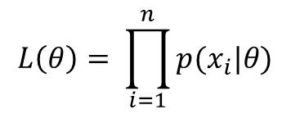# What is Likelihood Function in Data Science and Machine Learning?

I will try to explain Likelihood Function in very clear and simple terms. Likelihood Function in Machine Learning and Data Science is the joint probability distribution(jpd) of the dataset given as a function of the parameter.

Think of it as the probability of obtaining the observed data given the parameter values.

We would now define Likelihood Function for both discreet and continuous distributions:

For discreet distributions:

Assuming X is a discreet random variable (X can take value x)

Let the probability mass function (pmf) of x be p

Let the parameter of the distribution be θ

Then the likelihood function is simply given as:

##### L(θ | x) = p(x)

This is a function of θ.

L is the likelihood function of θ given x, the value of the random variable X.

Also, the probability that the random variable X takes the value x for the parameter θ is given as P(X = x | θ)

For continuous distributions:

Similarly, for continuous distribution, let X be a random variable can can take a value x.

X has a density function of f that depend on the parameter θ.

Then the function:

##### L(θ | x) = f(x)

for the parameter θ is the likelihood function of θ given x

#### A Closer Look at Likelihood Function

Let’s approach the definition from another way. Maybe, it’ll be clearer for you.

Assuming a set of observations X = x1, x2, …, xn that has a joint probability density function given by p(x1, x2, … , xn | θ)

Then the likelihood function  is given by

L(θ) = L(θ | x1, x2, …, xn) = p(x1, x2, …, xn |θ)

In this case, x1, x2,…, xn is fixed

Log Likelihood

It is generally easier to find the natural logarithm of the likelihood function. This is known as the log-likelihood and is given by:

log L(θ)

#### Properties of Likelihood Function

The likelihood function(lf) is a function is function of the parameter θ

The likelihood function is different from the probability density function

If the data is independent and identically distributed(iid), then the likelihood is given by:The likelihood function is defined up to some constant proportionality

It is used in estimation to generate estimators for example maximum likelihood estimation and for Bayesian inference.#### kindsonthegenius

Kindson Munonye is currently completing his doctoral program in Software Engineering in Budapest University of Technology and Economics

View all posts by kindsonthegenius →